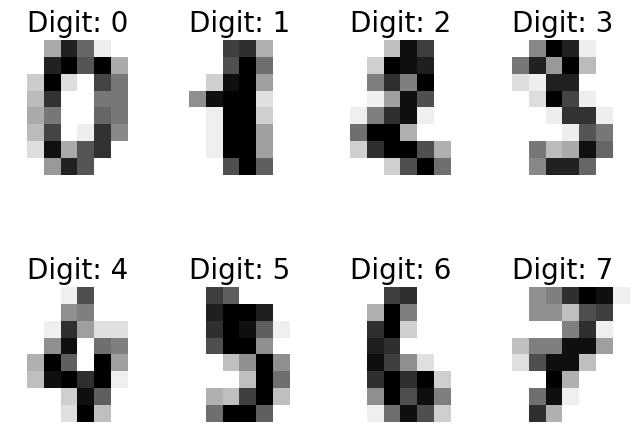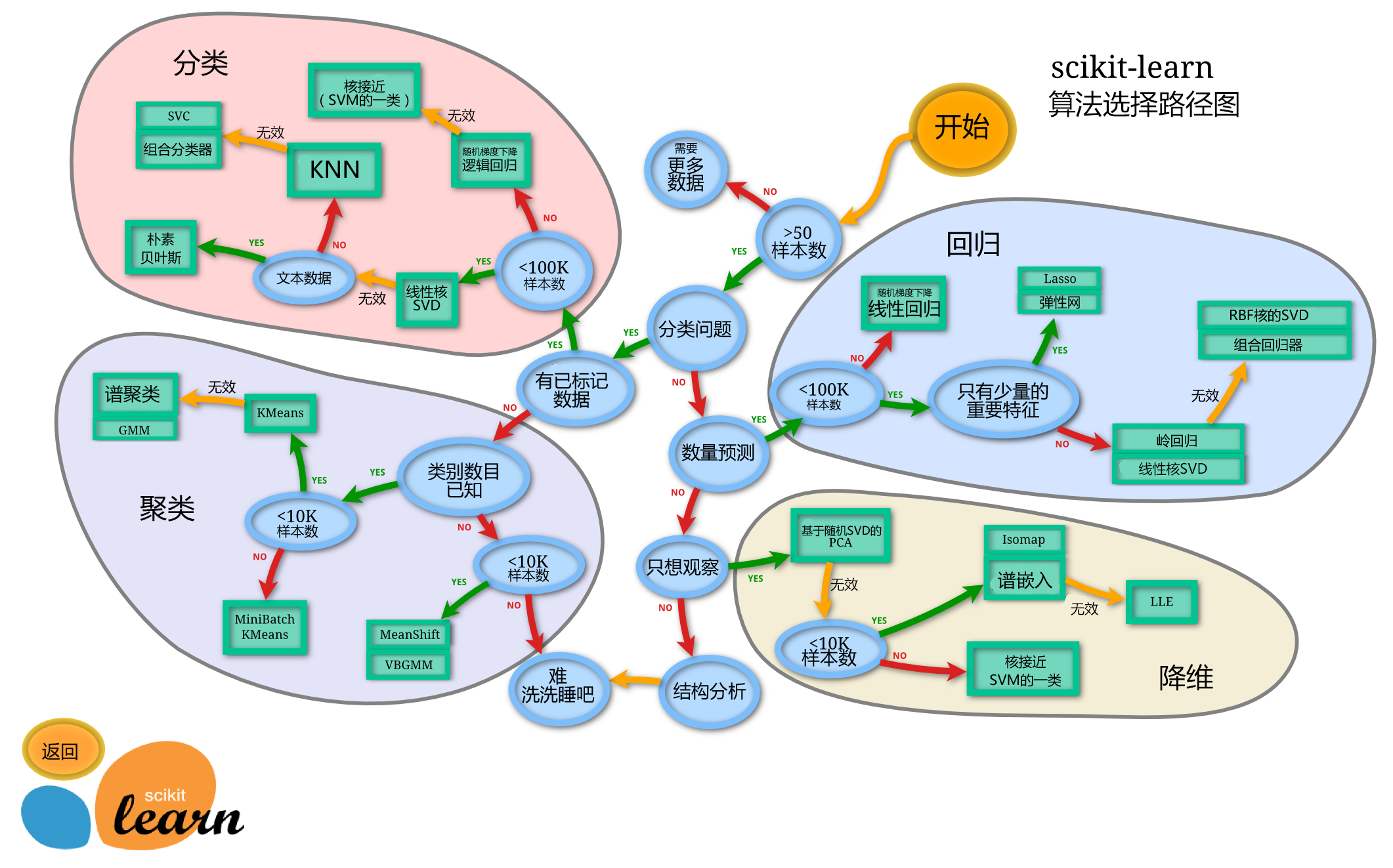# 机器学习的分类

1. 数据有标记——有监督学习；
2. 数据无标记——无监督学习。

# Python机器学习环境

• Python
• IPython(即Jupyter Notebook)
• Numpy
• Scipy
• Pandas
• Matplotlib
• scikit-learn

## IPython常用命令

• %run [hello.py] hello.py 是当前工作目录下的一个文件，该命令在一个空命名空间里运行该文件，并将文件里定义的全局变量和函数就会自动引用到当前 IPython空间中。
• %timeit [代码] 评估代码运行的效率（时间）
• %who %whos 查看当前环境的变量列表
• %matplotlib inline 使 matplotlib 的的图片直接画在网页上

## scikit-learn简介

scikit-learn是一个开源机器学习库。

# 机器学习的步骤

## 数据的采集和标记

from sklearn import datasets
print(digits)


%matplotlib inline
from matplotlib import pyplot as plt
images_and_labels = list(zip(digits.images, digits.target))
plt.figure(figsize=(8, 6), dpi=100)
for index, (image, label) in enumerate(images_and_labels[:8]):
plt.subplot(2, 4, index + 1)
plt.axis('off')
plt.imshow(image, cmap=plt.cm.gray_r, interpolation='nearest')
plt.title('Digit: %i' % label, fontsize=20)## 特征选择

print(" shape of raw image data: {0}".format(digits.images.shape))
print(" shape of data: {0}".format(digits.data.shape))

 shape of raw image data: (1797, 8, 8)
shape of data: (1797, 64)


## 模型训练

from sklearn.model_selection import train_test_split
Xtrain, Xtest, Ytrain, Ytest = train_test_split(digits.data,
digits.target,
test_size=0.8,
random_state=2)


# 使用支持向量机来训练模型
from sklearn import svm
clf = svm.SVC(gamma=0.001, C=100.)
clf.fit(Xtrain, Ytrain)

SVC(C=100.0, cache_size=200, class_weight=None, coef0=0.0,
decision_function_shape='ovr', degree=3, gamma=0.001, kernel='rbf',
max_iter=-1, probability=False, random_state=None, shrinking=True,
tol=0.001, verbose=False)

clf.score(Xtrain, Ytrain)

1.0


## 模型测试

clf.score(Xtest, Ytest)

0.9791376912378303


#查看预测的情况
fig, axes = plt.subplots(4, 4, figsize=(8, 8))
Ypred = clf.predict(Xtest)
for i, ax in enumerate(axes.flat):
ax.imshow(Xtest[i].reshape(8, 8),
cmap=plt.cm.gray_r,
interpolation='nearest')
ax.text(0.05,
0.05,
str(Ypred[i]),
fontsize=32,
transform=ax.transAxes,
color='green' if Ypred[i] == Ytest[i] else 'red')
ax.text(0.8,
0.05,
str(Ytest[i]),
fontsize=32,
transform=ax.transAxes,
color='black')
ax.set_xticks([])
ax.set_yticks([])## 模型保存与加载

#保存模型参数
import joblib
joblib.dump(clf, 'digits_svm.pkl')

['digits_svm.pkl']

#导入模型参数，直接进行预测
Ypred = clf.predict(Xtest)
clf.score(Xtest, Ytest)

0.9791376912378303


# scikit-learn一般性原理

## 评估模型对象

scikit-learn里的所有算法都以一个评估模型对象来对外提供接口。上面例子里的 svm.SVC()函数返回的就是一个支持向量机评估模型对象。创建评估模型对象时，可以指定不同的参数，这个称为评估对象参数，评估对象参数直接影响评估模型训练时的效率以及准确性。

## 模型接口

scikit-learn所有的评估模型对象都有 fit() 这个接口，这是用来训练模型的接口。针对有监督的机器学习（如上面的例子），使用 fit(x, y) 来进行训练，其中 y是标记数据。针对无监督的机器学习算法，使用 fit(x) 来进行训练，因为无监督机器学习算法的数据集是没有标记的，不需要传入 y。

## 模型选择